# High School Chemistry : Using SI Units

## Example Questions

### Example Question #1 : Units

What unit will be used to denote temperature in thermochemistry?

Kelvin

Celsius or Kelvin

Joules

Fahrenheit

Celsius

Kelvin

Explanation:

Temperature is the measure of the average kinetic energy of a system. Kelvin is the SI unit for temperature, and must be used because it is the only scale that does not have any negative numbers possible. At zero Kelvin (absolute zero) and there is absolutely no movement in the system, down to the atomic level.

While temperature is a means of measuring energy in a system, actual heat energy has the unit Joules.

### Example Question #1 : High School Chemistry

Which of the following measurements contains 4 significant figures?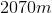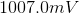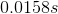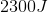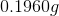Explanation:

1. Any digit with a value 1-9 is always a significant figure

2. Any 0 between two digits 1-9 is always significant

3. If a number is greater than 1 and does not have a decimal point a 0 at the end is not significant because it is a tailing zero

4. If a number is less than 1 any 0 before a digit 1-9 is not significant because it is a leading zero

5. If there is a decimal point in any number, any 0 that follows a non-zero digit is always significant.

Thus,has 4 significant figures. The first 0 is not significant because of rule 4. The digits 1, 9, and 6 are always significant (rule 1) and the last 0 is also significant because there is a decimal point and that 0 follows a non-zero digit (rule 5).

### Example Question #3 : Units

Which has the larger unit listed first?

Centigram and decigram

Kilojoule and megajoule

Microsecond and nanosecond

Decaliter and kiloliter

Picometer and millimeter

Microsecond and nanosecond

Explanation:

The following prefixes are ranked in order from largest to smallest with the power of 10 that the unit represents: peta (P)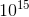> tera (T)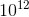> giga (G)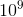> mega (M)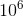> kilo (k)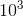> hecta (H)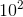> deca (D)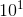> unit > deci (d)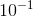> centi (c)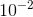> milli (m)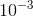> micro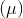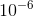> nano (n)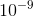> pico (p)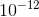> femto (f)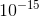. Thus a microsecond is one thousand times longer than a nanosecond.

### Example Question #1 : High School Chemistry

The measurement of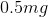is equal to which of the following?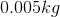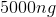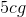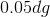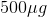Explanation:

Units in the metric system can be converted using the correct factor of 10.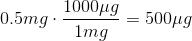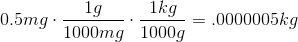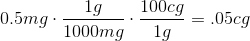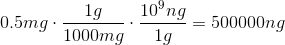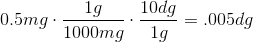### Example Question #5 : Units

A certain metal is weighed on a balance in a weighing boat. Before the metal is added, the mass of the weigh boat is recorded at. The final mass of the weigh boat and the metal is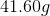. The metal is added to a graduated cylinder filled with water to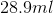. After the metal is added to the water the graduated cylinder reads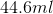.

What is the density of the metal?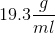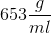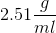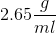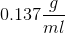Explanation:

Density is equal to the mass of the object divided by the volume.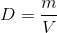The mass of the metal is figured out by taking the mass of the metal and the weighboat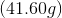and subtracting the weighboat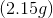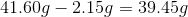The volume of the water is calculated by the displacement of the water so take the final volume and subtract the initial volume.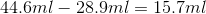The density of the metal is calculated by taking the mass divided by the volume.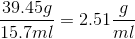after rounding to 3 significant figures.

### Example Question #1 : High School Chemistry

An object is measured to be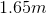tall. How tall is the object in inches?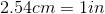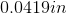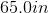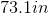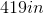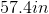Explanation:

You must use equalities and dimensional analysis to solve for the correct answer: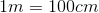Each equality allows you to write a conversion factor 2 ways:

Example:can be written as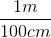and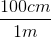To cancel out units if there is a unit on top it must be cancelled out by the same unit on the bottom.

To solve you must cancel out units until you get to the proper unit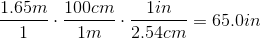### All High School Chemistry Resources